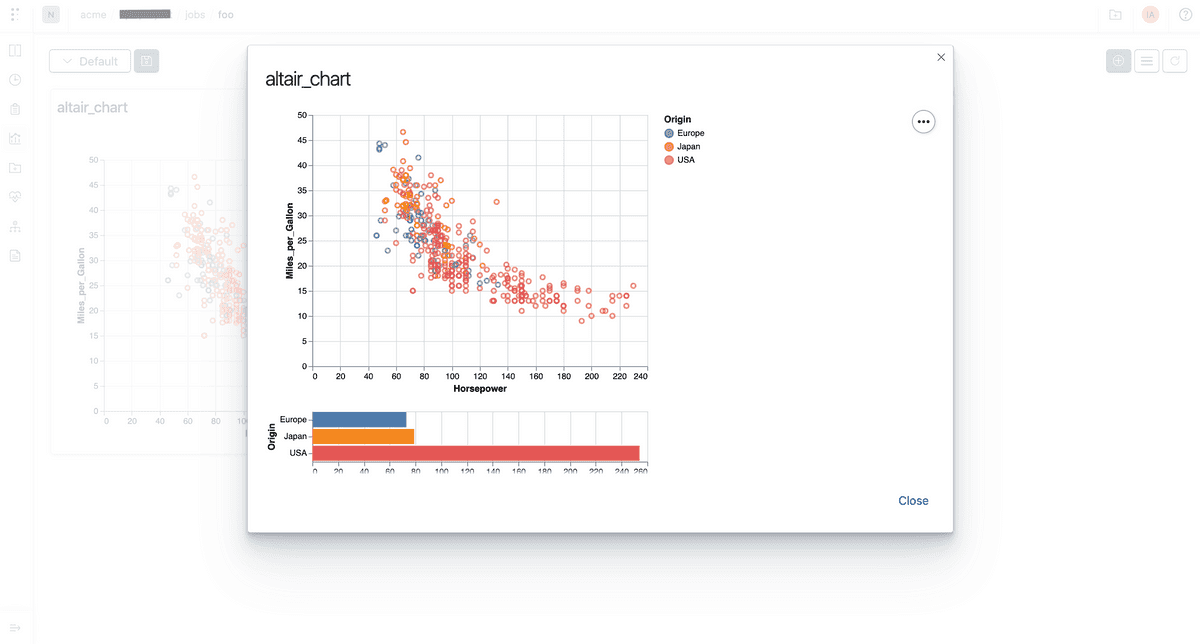## Overview

Polyaxon comes with a powerful and customizable visualization system for driving insights in the dashboard or programmatically.

Oftentimes users have advance use cases that are not supported by the platform, or they need to integrate visualizations generated by external systems.

Polyaxon can consume any chart specification generated by these libraries:

• Matplotlib
• Plotly
• Bokeh
• Altair/Vega

## Matplotlib

You can log charts generated by Matplotlib directly to Polyaxon.

Polyaxon supports two methods for logging matplotlib figures:

• Logging figures as images
• Parsing the figures to interactive charts

### Matplotlib static figures

``````from polyaxon import tracking

figure = ...

tracking.init(...)

tracking.log_mpl_image(figure, name="my-figure", step=12)``````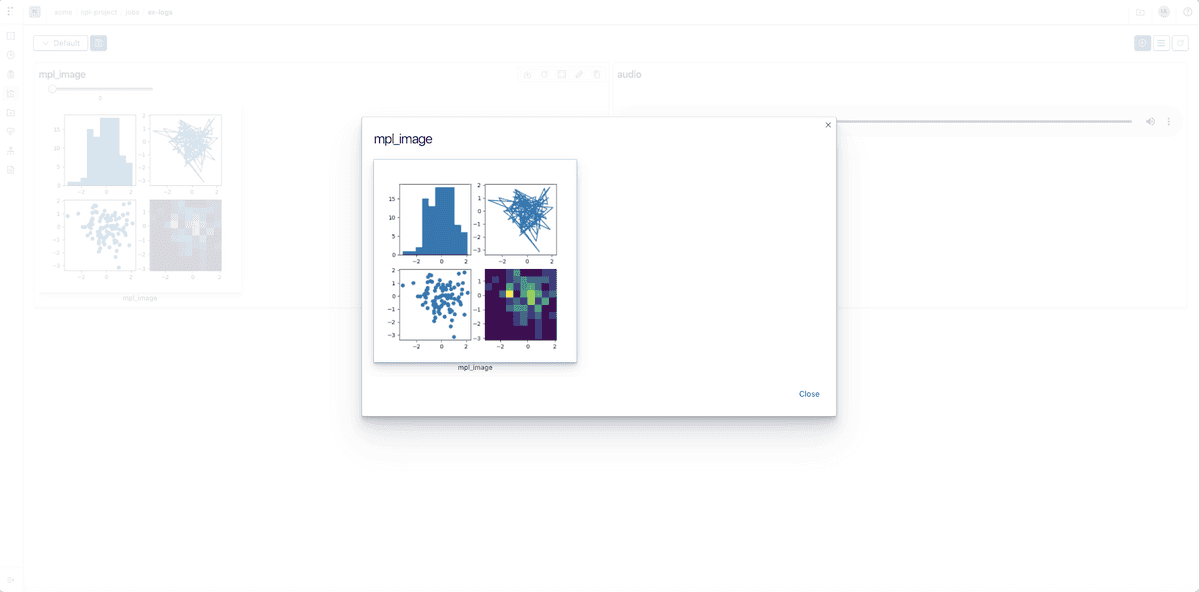### Matplotlib interactive figures

``````from polyaxon import tracking

figure = ...

tracking.init(...)

tracking.log_mpl_plotly_chart(figure=figure, name="my-figure", step=12)``````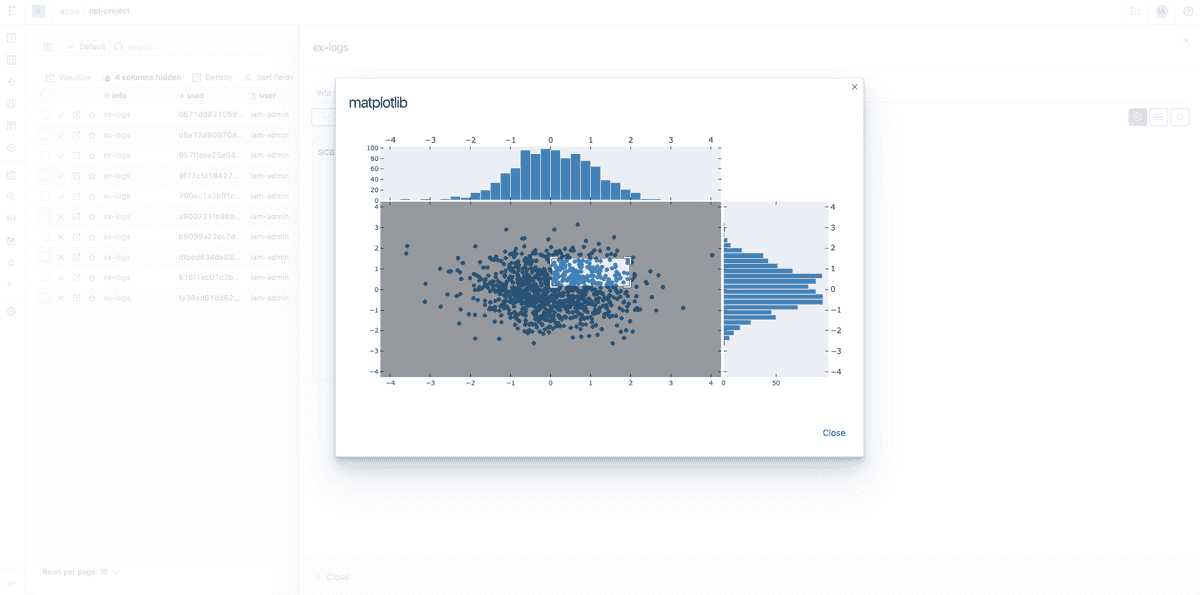## Plotly

You can log charts generated by Plotly directly to Polyaxon.

``````from polyaxon import tracking

figure = ...

tracking.init(...)

tracking.log_plotly_chart(figure=figure, name="my-figure", step=12)``````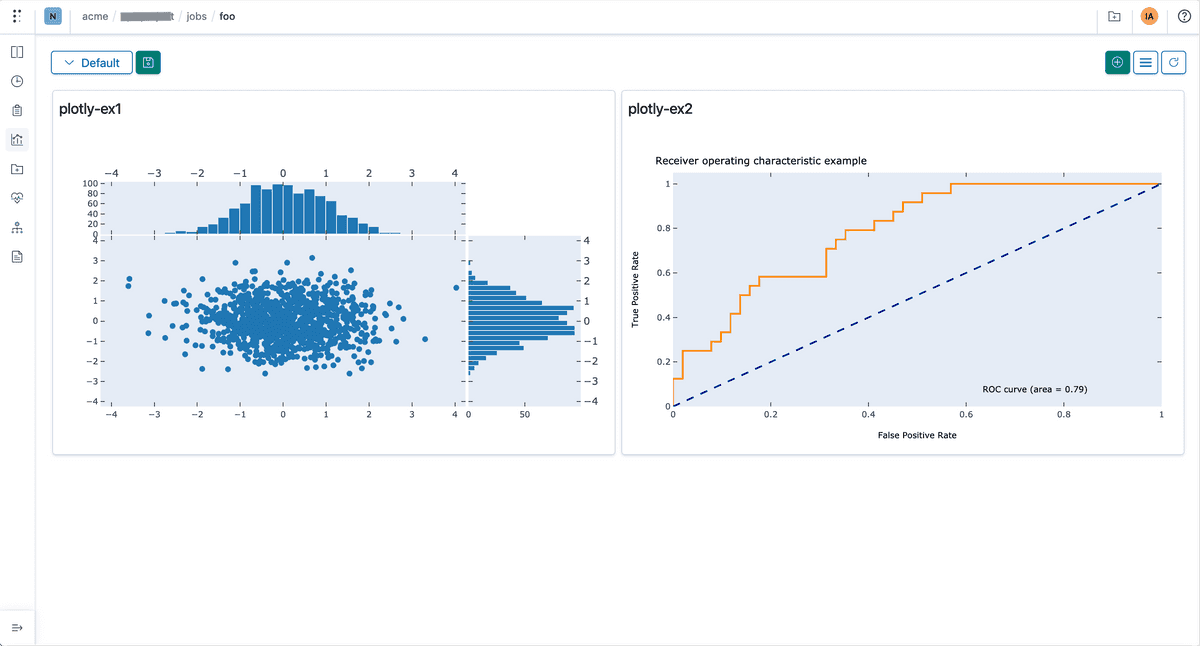## Bokeh

You can log charts generated by Bokeh directly to Polyaxon.

``````from polyaxon import tracking

figure = ...

tracking.init(...)

tracking.log_bokeh_chart(figure=figure, name="my-figure", step=12)``````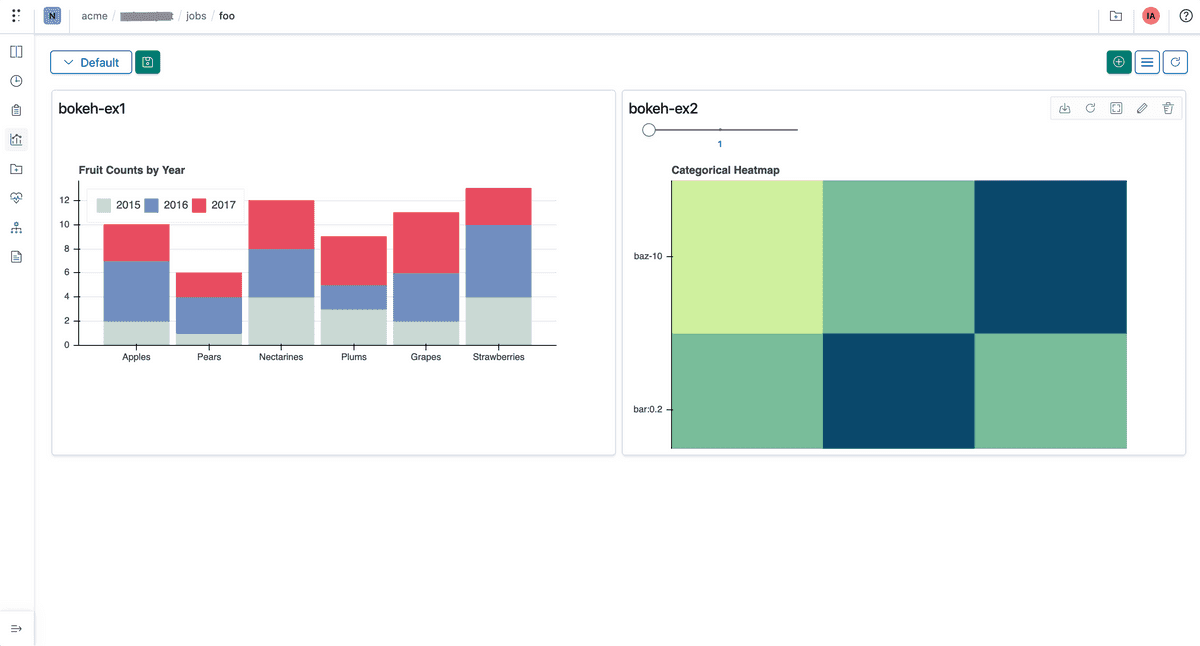## Altair

You can log charts generated by Altair or vega-light directly to Polyaxon

``````from polyaxon import tracking

figure = ...

tracking.init(...)

tracking.log_altair_chart(figure=figure, name="my-figure", step=12)``````